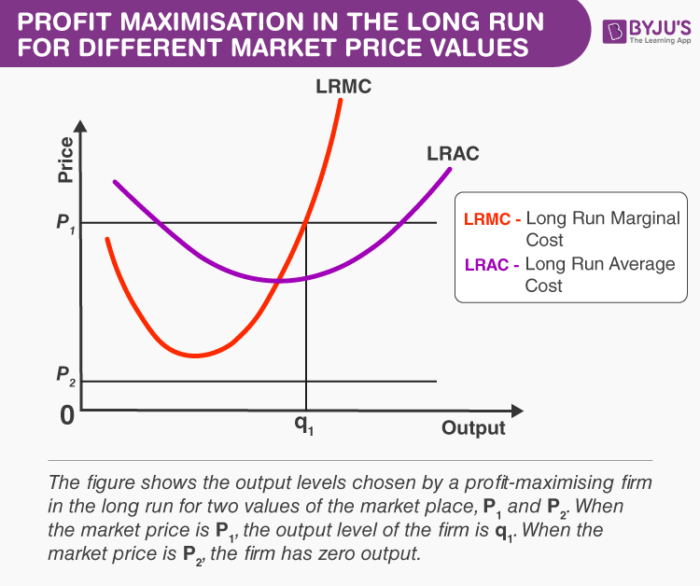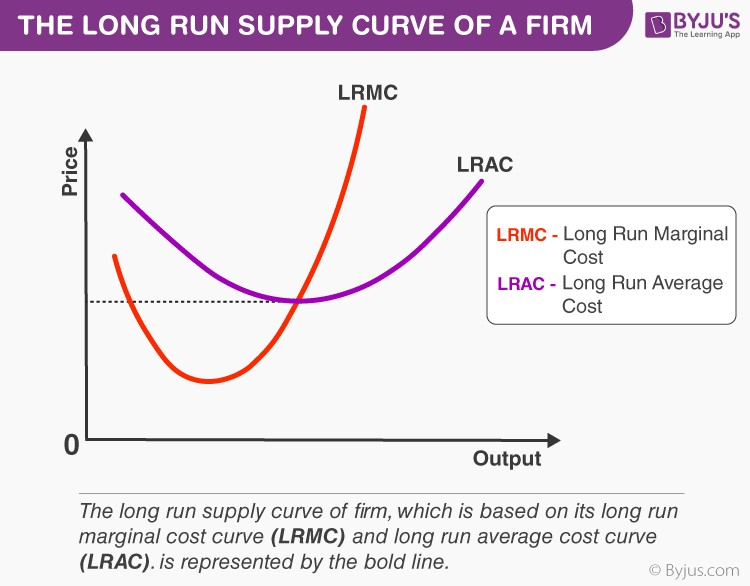# Long Run Supply Curve of a Firm

Let us derive an enterprises long-run supply curve. While dividing the  short-run curve, we divide the derivation into two parts. We first decide the enterprises profit-maximising output degree when the market cost price is greater than or equivalent to the minimum (long-run) AC.

We decide the enterprises profit-maximising output degree when the market cost price is less than the minimum (long-run) AC.

## Case 1: Price Greater Than or Equal to the Minimum LRAC

Assume that the market cost price is p1, which surpasses the minimum LRAC. Upon equating p1 with LRMC on the increasing part of the LRMC curve, we procure the output degree q1.

Also, note that the LRAC at q1 does not surpass the market cost price, p1. Hence, all the three conditions  in section 3 are satisfied at q1. Thus, when the market cost price is p1, the enterprises supplies in the long-run become equal to q1.Also Read: Short Run Supply Curve of a Firm

## Case 2: Price Less Than the Minimum LRAC

Assume that the market cost price is p2, which is less than the minimum LRAC. If a profit-maximising enterprise manufactures a positive output in the long-run, the market cost price, p2, must be greater than or equal to the LRAC at that output degree.

In other words, the enterprise cannot supply a positive output. So, when the market cost price is p2, the enterprise has zero output. Combining cases 1 and 2, we come to an important conclusion.

An enterprises long-run supply curve is the increasing part of the LRMC curve from and above the minimum LRAC, together with the zero output for all the cost prices less than the minimum LRAC.This was the concept of the long-run supply curve of a firm. To learn more such interesting concepts of commerce, keep visiting our website.